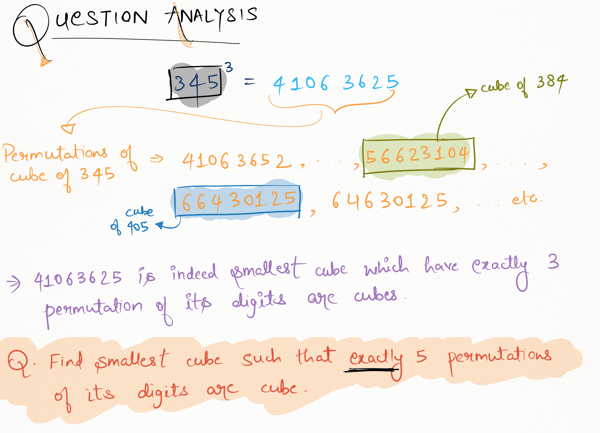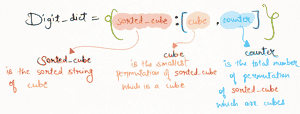Related Tags

python
project euler
communitycreator

# Project Euler 62: Cubic permutations

Armaan Nougai

### Problem statement

The permutations of digits of the cube of 345, 41063625, can be the cubes of 384 and 405.

Find the smallest cube such that exactly five of its permutations are cubes.### Solution approach

Since we need to count how many cubes there are in permutations of a particular set of digits, we will store every distinct set of digits, along with their smallest cubic permutation and the total cubic permutations until we encounter the first/smallest set of digits that have exactly 5 cubic permutations. We will store this information in mapped objects, i.e., Dictionary in Python.

13451,31451,11453… are all permutations of a particular set of digits, i.e., {1,1,3,4,5}.### Code explanation

We will run a while loop for n times, starting from 1, until any of the keys have the counter in Digit_dict == 5.

#### Variables

1. n_cube = cube of n, i.e., $n^3$.

2. sorted_cube = the sorted string of n_cube.

3. cube = the n whose cube is the smallest cubic permutation of sorted_cube.

4. counter = the number of cubic permutations of sorted_cube.

5. Digit_dict = a Python dictionary, where:

i. keys are sorted_cube.

ii. values are [ cube ,counter ].

#### Conditions

1. if sorted_cube is already in Digit_dict keys:

sorted_cube is already in Digit_keys. This means n_cube is just a permutation of sorted_cube. Therefore, we will simply increment counter in Digit_keys for sorted_cube by 1.

2. else:

sorted_cube is not in Digit_keys. This means n_cube is not a permutation of any previous cubes. Therefore, we will add this sorted_cube in Digit_dict.

Digit_dict = dict()

n = 1
while True:
n_cube = n**3
sorted_cube = ''.join(sorted(str(n_cube)))

if sorted_cube in list(Digit_dict.keys()):

Digit_dict[sorted_cube] = [Digit_dict[sorted_cube],Digit_dict[sorted_cube]+1]
else:

Digit_dict.update({sorted_cube : [n_cube,1]})

if Digit_dict[sorted_cube] == 5:

print(Digit_dict[sorted_cube])
break
n+=1



RELATED TAGS

python
project euler
communitycreator

CONTRIBUTOR

Armaan Nougai
RELATED COURSES

View all Courses

Keep Exploring

Learn in-demand tech skills in half the time